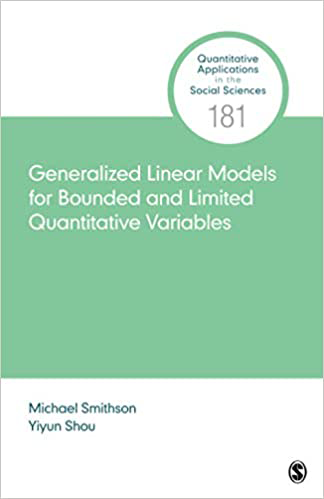# Generalized Linear Models for Bounded and Limited Quantitative Variables

Generalized Linear Models for Bounded and Limited Quantitative Variables provides a focused discussion on the theoretical and applied aspects of modeling outcomes with natural boundaries, such as proportions, and outcomes subjected to censoring or truncation. The authors introduce models that use appropriate distributions for various types of bounded outcomes, such as beta regression for outcomes bounded between 0 and 1. They also introduce models such as Tobit models that account for censoring and models that can account for excess observations at boundaries. Researchers and students who have some familiarity with generalized linear models will find this book to be a great resource when they are ready to model bounded and limited dependent variables.

The book’s companion website provides annotated code in Stata, SAS, and R, as well as the datasets needed to reproduce the examples in the book. These resources make it easy for readers to learn not only the theory behind these models but also the practical aspects of fitting the models and interpreting the results.

INTRODUCTION AND OVERVIEW
Overview of This Book
The Nature of Bounds on Variables
The Generalized Linear Model
Examples

MODELS FOR SINGLY BOUNDED VARIABLES
GLMs for Singly Bounded Variables
Model Diagnostics
Treatment of Boundary Cases

MODELS FOR DOUBLY BOUNDED VARIABLES
Doubly Bounded Variables and “Natural” Heteroscedasticity
The Beta Distribution: Definition and Properties
Modeling Location and Dispersion
Estimation and Model Diagnostics
Treatment of Cases at the Boundaries

QUANTILE MODELS FOR BOUNDED VARIABLES
Introduction
Quantile Regression
Distributions for Doubly Bounded Variables With Explicit Quantile Functions
The CDF-Quantile GLM

CENSORED AND TRUNCATED VARIABLES
Types of Censoring and Truncation
Tobit Models
Tobit Model Example
Heteroscedastic and Non-Gaussian Tobit Models

EXTENSIONS AND CONCLUSIONS
Extensions and a General Framework
Absolute Bounds and Censoring
Multilevel and Multivariate Models
Bayesian Estimation and Modeling
Roads Less Traveled and the State of the ArtAuthor: Michael Smithson and Yiyun Shou
ISBN-13: 978-1-54433-453-0